Next: Index based algorithms Up: Other Partitioning Algorithms Previous: Global optimization algorithms

### Reducing the bandwidth of the matrix

As we have seen in the discussion of the RSB algorithm (equation (6)), an undirected graph can be uniquely represented by an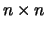matrix, where the entry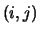is nonzero if vertices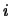and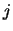are connected by an edge and zero if the two vertices are not connected. A good bisection of the graph is equivalent to an ordering, through symmetric permutation, of the matrix such that the resulting matrix has very few nonzero off-diagonal entries corresponding to the two principal diagonal blocks of size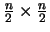. This is because the off-diagonal entries correspond to the edges between the two subdomains. Although such an ordering algorithm is not readily available, there are a number of algorithms that order the matrix to minimize its bandwidth. A partitioning algorithm  was suggested which orders the vertices of the graph using matrix ordering algorithms, and partitions the graph by taking the first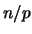vertices as the first subdomain, etc.. The algorithm is inexpensive and can usually result in a smaller number of neighbors for each processor. The edge-cut can be improved if the algorithm is used recursively as a bisection algorithm. Nonetheless in terms of edge-cut its is not as good as algorithms such as the RSB or the greedy algorithm .Next: Index based algorithms Up: Other Partitioning Algorithms Previous: Global optimization algorithms

2000-03-21